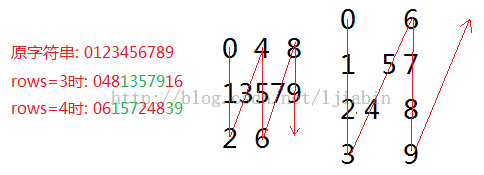# 凌云的博客

## LeetCode 算法题 6. Z 字形变换

### 题目

``````L   C   I   R
E T O E S I I G
E   D   H   N
``````

``````string convert(string s, int numRows);
``````

``````输入: s = "LEETCODEISHIRING", numRows = 3

``````

``````输入: s = "LEETCODEISHIRING", numRows = 4

``````

``````L     D     R
E   O E   I I
E C   I H   N
T     S     G
``````

### 解法1``````class Solution {
public:
string convert(string s, int numRows) {
const int size = s.size();
if (numRows == 1) {
return s;
}

int distance, distance2;
string ret;
for (int i = 0; i < numRows; ++i) {
distance = 2 * (numRows - 1);
distance2 = i ? distance - (2 * i) : 0;

for (int j = i; j < size; j += distance) {
ret.push_back(s[j]);
if (distance2 && j + distance2 < size) {
ret.push_back(s[j + distance2]);
}
}
}

return ret;
}
};
``````